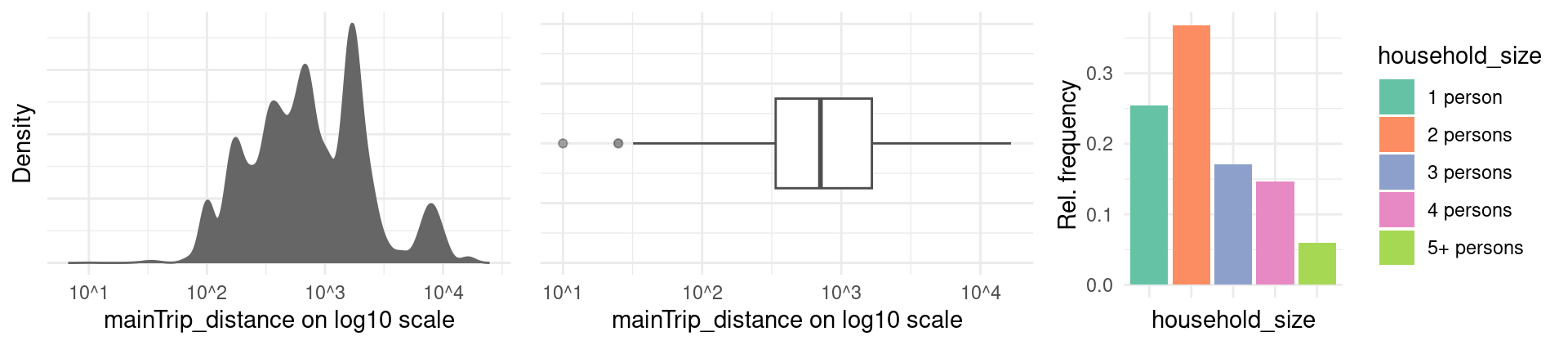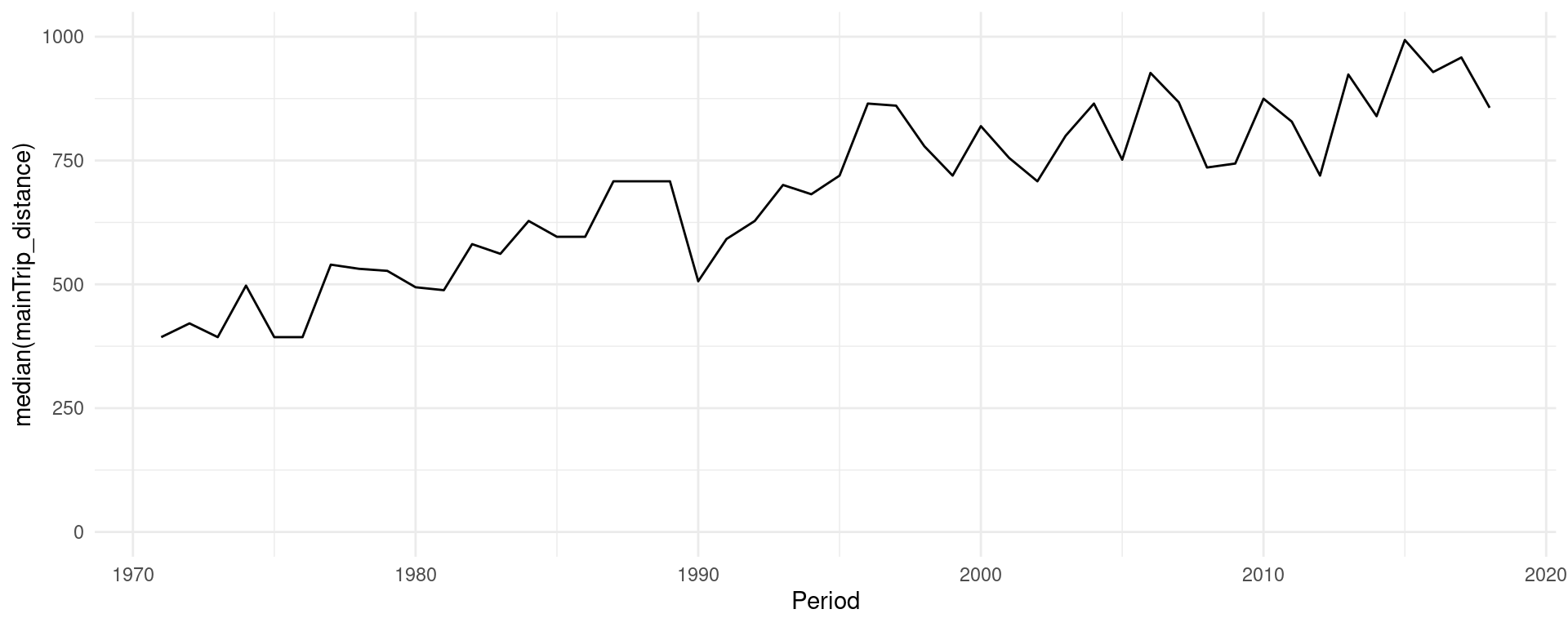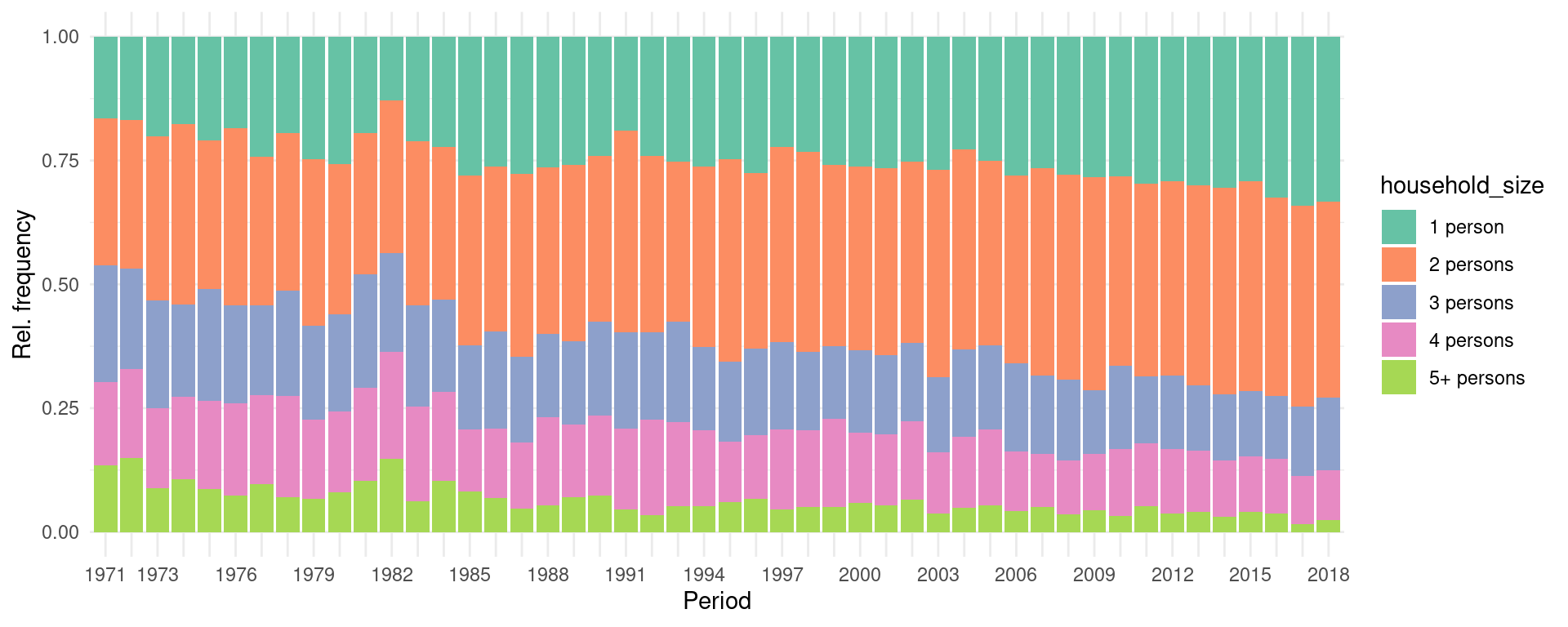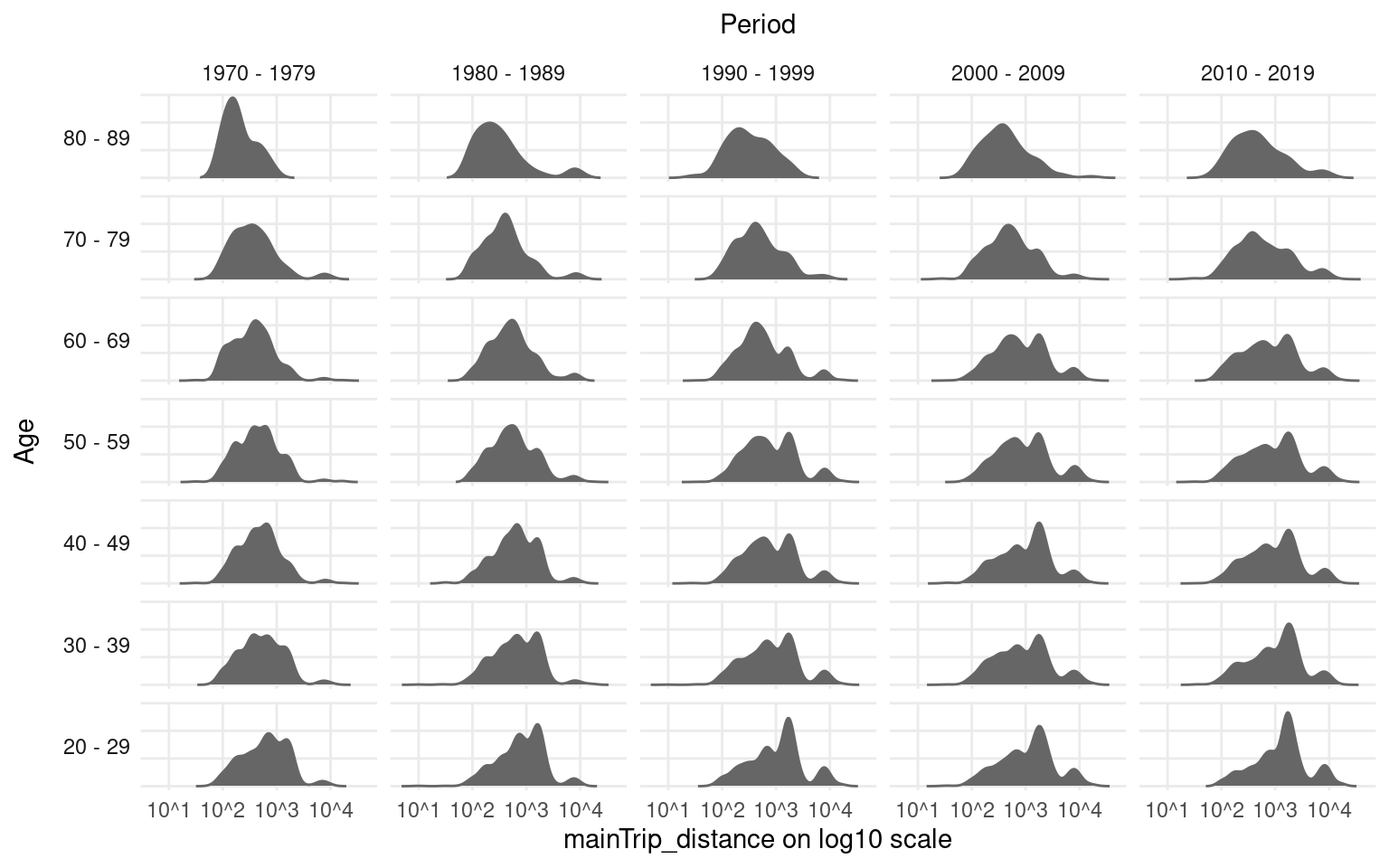This document gives an overview of the functionality provided by the R package `APCtools`.

Age-Period-Cohort (APC) analysis is used to disentangle observed trends (e.g. of social, economic, medical or epidemiological data) to enable conclusions about the developments over three temporal dimensions:

• Age, representing the developments associated with chronological age over someones life cycle.
• Period, representing the developments over calendar time which affect all age groups simultaneously.
• Cohort, representing the developments observed over different birth cohorts and generations.

The critical challenge in APC analysis is that these main components are linearly dependent: \[ cohort = period - age \]

Accordingly, flexible methods and visualization techniques are needed to properly disentagle observed temporal association structures. The `APCtools` package comprises different methods that tackle this problem and aims to cover all steps of an APC analysis. This includes state-of-the-art descriptive visualizations as well as visualization and summary functions based on the estimation of a generalized additive regression model (GAM). The main functionalities of the package are highlighted in the following.

For details on the statistical methodology see Weigert et al. (2021) or our corresponding research poster. The hexamaps (hexagonally binned heatmaps) are outlined in Jalal & Burke (2020).

Before we start, let’s load the relevant packages for the following analyses.

``````library(APCtools)
library(dplyr)    # general data handling
library(mgcv)     # estimation of generalized additive regression models (GAMs)
library(ggplot2)  # data visualization
library(ggpubr)   # arranging multiple ggplots in a grid with ggarrange()

# set the global theme of all plots
theme_set(theme_minimal())``````

## Example data

APC analyses require long-term panel or repeated cross-sectional data. The package includes two exemplary datasets on the travel behavior of German tourists (dataset `travel`) and the number of unintentional drug overdose deaths in the United States (`drug_deaths`). See the respective help pages `?travel` and `?drug_deaths` for details.

In the following, we will use the `travel` dataset to investigate if travel distances of the main trip of German travelers mainly change over the life cycle of a person (age effect), macro-level developments like decreasing air travel prices in the last decades (period effect) or the generational membership of a person, which is shaped by similar socialization and historical experiences (cohort effect).

``data(travel)``

## Descriptive visualizations

Different functions are available for descriptively visualizing observed structures. This includes plots for the marginal distribution of some variable of interest, 1D plots for the development of some variable over age, period or cohort, as well as density matrices that visualize the development over all temporal dimensions.

### Marginal distribution of one variable

The marginal distribution of a variable can be visualized using `plot_density`. Metric variables can be plotted using a density plot or a boxplot, while categorical variables can be plotted using a bar chart.

``````gg1 <- plot_density(dat = travel, y_var = "mainTrip_distance", log_scale = TRUE)
gg2 <- plot_density(dat = travel, y_var = "mainTrip_distance", log_scale = TRUE,
plot_type = "boxplot")
gg3 <- plot_density(dat = travel, y_var = "household_size")

ggpubr::ggarrange(gg1, gg2, gg3, nrow = 1)``````### 1D: One variable against age, period or cohort

Plotting the distribution of a variable against age, period or cohort is possible with function `plot_variable`. The distribution of metric and categorical variables is visualized using boxplots or line charts (see argument `plot_type`) and bar charts, respectively. The latter by default show relative frequencies, but can be changed to show absolute numbers by specifying argument `geomBar_position = "stack"`.

``````plot_variable(dat = travel, y_var = "mainTrip_distance",
apc_dimension = "period", plot_type = "line", ylim = c(0,1000))````````plot_variable(dat = travel, y_var = "household_size", apc_dimension = "period")``### 2D: Density matrices

To include all temporal dimensions in one plot, `APCtools` contains function `plot_densityMatrix`. In Weigert et al. (2021), this plot type was referred to as ridgeline matrix when plotting multiple density plots for a metric variable. The basic principle of a density matrix is to (i) visualize two of the temporal dimensions on the x- and y-axis (specified using the argument `dimensions`), s.t. the third temporal dimension is represented on the diagonals of the matrix, and (ii) to categorize the respective variables on the x- and y-axis in meaningful groups. The function then creates a grid, where each cell contains the distribution of the selected `y_var` variable in the respective category.

By default, age and period are depicted on the x- and y-axis, respectively, and cohort on the diagonals. The categorization is defined by specifying two of the arguments `age_groups`, `period_groups` and `cohort_groups`.

``````age_groups    <- list(c(80,89),c(70,79),c(60,69),c(50,59),
c(40,49),c(30,39),c(20,29))
period_groups <- list(c(1970,1979),c(1980,1989),c(1990,1999),
c(2000,2009),c(2010,2019))

plot_densityMatrix(dat              = travel,
y_var            = "mainTrip_distance",
age_groups       = age_groups,
period_groups    = period_groups,
log_scale        = TRUE)``````To highlight the effect of the variable depicted on the diagonal (here: cohort), different diagonals can be highlighted using argument `highlight_diagonals`.

``````plot_densityMatrix(dat                 = travel,
y_var               = "mainTrip_distance",
age_groups          = age_groups,
period_groups       = period_groups,
highlight_diagonals = list("born 1950 - 1959" = 8,
"born 1970 - 1979" = 10),
log_scale           = TRUE)``````